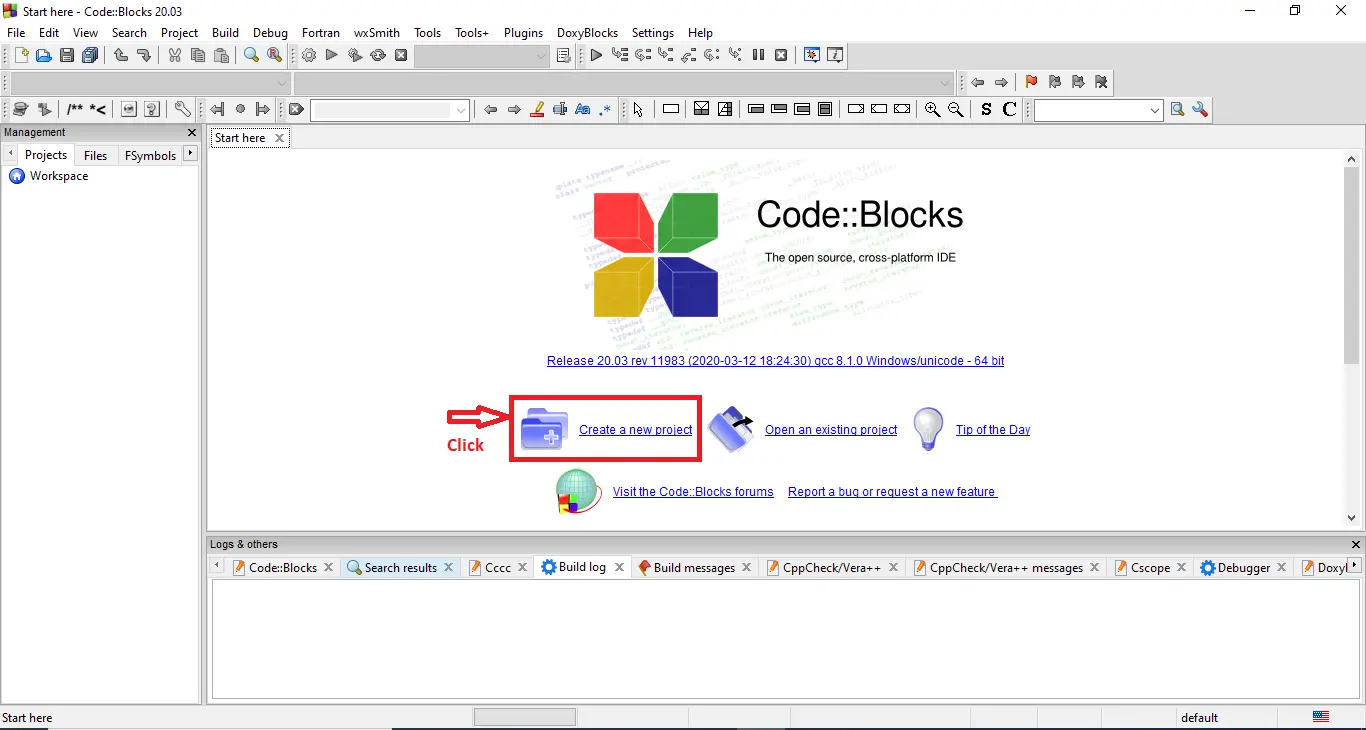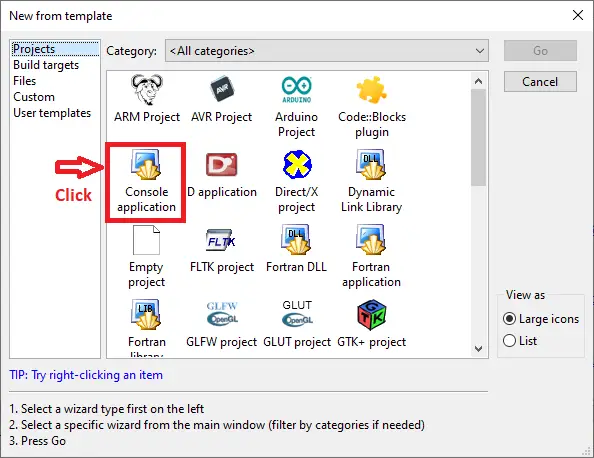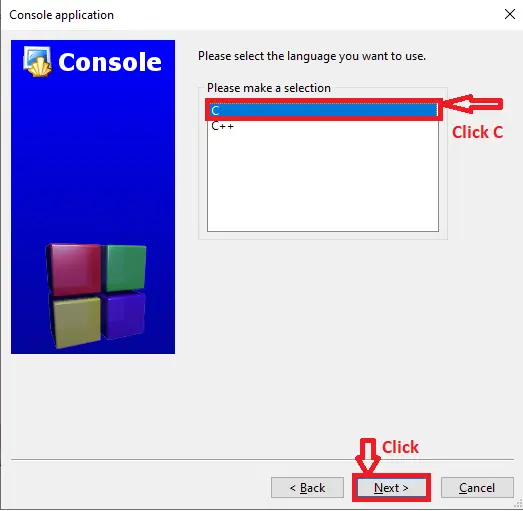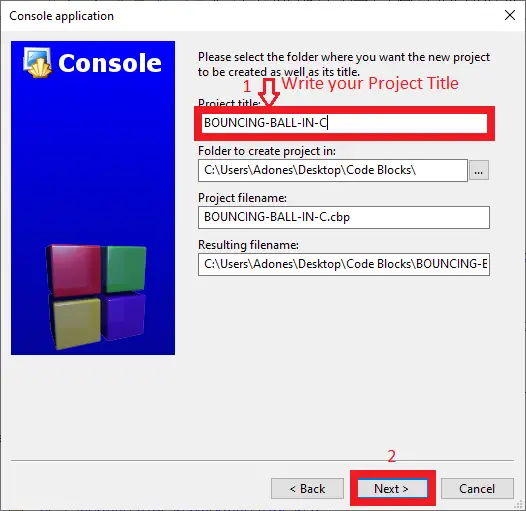# Bouncing Ball in C with Source Code

## Bouncing Ball in C with Source Code

This Bouncing Ball Game Project in C teaches you how to create a simple bouncing ball game using computer programs. In the console interface, the ball keeps moving. It reverses course when it comes into contact with the border. The ball is caught by the control stick, and the score is increased by one. The game is over if the ball is not caught.

Controlling the ball’s movement direction via a variable is crucial to the effect of the ball movement. This variable changes depending on the boundary encountered and its current value, therefore the direction of flight is in the exact opposite direction.

Then, to conclude the game, set the ball to only hit the lower boundary where the stick is positioned, rather than touching the lower boundary where the stick is located.

Bouncing Ball in C with Source Code...
Bouncing Ball in C with Source Code | C Language Projects with Source Code

Bouncing Ball Game Code in C Language it include a free Download Source Code. Simply locate the downloadable source code below and click the “Download Now” button. To start creating a Calculator in C Language, make sure that you have a Code Blocks or any platform of C installed in your computer.

## Bouncing Ball in C Steps on How to Create a Project

Time needed: 5 minutes.

Here’s the step’s on how to create a Bouncing Ball in C with Source Code.

• Step 1: Create a new project.

First open the code blocks IDE and click “create a new project“.• Step 2: Choose console application.

Next, click the “console application” and after that click “next“.• Step 3: Choose C language.

Then , choose “C language” and click “next“.• Step 4: Name your project.

Lastly, name the project you’ve created and click “next” after that click “finish“.• Step 5: The actual code.

Finally, we will now start adding functionality to our C Framework by adding some functional codes.

## This Bouncing Ball in C was build and run under Code::Blocks IDE.

• This is the code to Define global variables in the system, the score board is for the number of squares eliminated, left and right for the boundary of keyboard.
```int hight,width;
int bouncingBall_X,bouncingBall_Y;
int ball_bouncing_vertical_x,ball_bouncing_vertical_y;
int pos_X,pos_Y;
int left,right;
int ball_number;
int blocking_X,blocking_Y;
int score_board;
```
• This is the function to hide the cursor(Bouncing Ball Game Project in C)
```void HideCursor()
{
CONSOLE_CURSOR_INFO cursor_info = {1, 0};
SetConsoleCursorInfo(GetStdHandle(STD_OUTPUT_HANDLE), &cursor_info);
}```
• Function to move the cursor to (x,y) position and clear screen function.
```void gotoxy(int x,int y)
{
HANDLE handle = GetStdHandle(STD_OUTPUT_HANDLE);
COORD pos;
pos.X = x;
pos.Y = y;
SetConsoleCursorPosition(handle,pos);
}```
• Function to display interface of the game such as output of the ball, output slider, output lower boundary, and output right margin.(Bouncing Ball Game Project in C)
```void show() //Display interface
{
gotoxy(0,0);
int i,j;
for(i=0;i<=hight;i++)
{
for(j=0;j<=width;j++)
{
if((i==bouncingBall_X) && (j==bouncingBall_Y))
printf("0");
else if((i==blocking_X)&& (j==blocking_Y))
printf("@");
else if(i==hight)
printf("-");
else if(j==width)
printf("|");
else if((i==hight-1)&&(j>left)&&(j<right))
printf("*");
else printf(" ");
}
printf("\n");
}
printf("Number of rebounds:%d\n",ball_number);
printf("The number of balls eliminated:%d\n",score_board);
}
```
• Function to moves the ball in the direction of speed, the ball hits the upper and lower boundaries, he ball hits the left and right boundaries, and the ball hits the slider.(Bouncing Ball Game Project in C)
```bouncingBall_X = bouncingBall_X + ball_bouncing_vertical_x;
bouncingBall_Y = bouncingBall_Y + ball_bouncing_vertical_y;

if((bouncingBall_X==0) || (bouncingBall_X==hight-2))
ball_bouncing_vertical_x=-ball_bouncing_vertical_x;
if((bouncingBall_Y==0) || (bouncingBall_Y==width-1))
ball_bouncing_vertical_y=-ball_bouncing_vertical_y;

if((blocking_X==bouncingBall_X) && (blocking_Y==bouncingBall_Y))
{
blocking_Y=rand()%width-1;
score_board++;
}```
• Function to change the color of the console.
`system("color 9f"); `